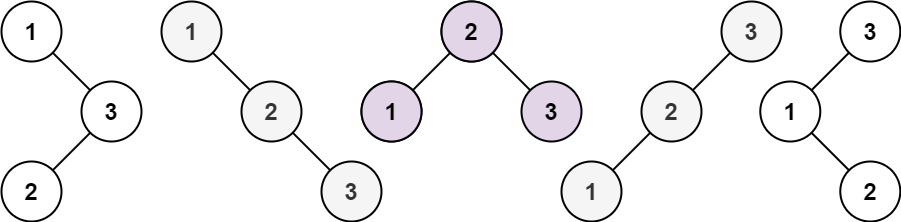# Nth Catalan Number

Hi Geeks, in this article we going to see about what are Catalan numbers, how to find nth Catalan number, by using Catalan number how we can find the Unique Binary Search trees.

``Catalan Numbers are: 1, 1, 2, 5, 14, 42, .....``

### Catalan Number Formation

``````C = 1
C = 1

C = CC + CC = (1 * 1) + (1 * 1) = 2

C = CC + C + C = (1 * 2) + (1 * 1) + (2 * 1) = 5

C = C + C + C + C=(1 * 5) + (1 * 2) + (2 * 1) + (5 * 1) = 14
.
.
.
C[N] = C[N-1] + C[N-2] + ... C[i][N-i-1] +.... + C[N-1]C``````

By using Catalan Numbers approach, here we will solve one problem from Leetcode – Unique Binary Search Trees.

Given an integer `n`, return the number of structurally unique BST’s (binary search trees) which has exactly `n` nodes of unique values from `1` to `n`.

Example 1:```Input: n = 3
Output: 5
```

Example 2:

```Input: n = 1
Output: 1
```

Constraints:

• `1 <= n <= 19`

## Solution

### Java Program

``````class Solution {
public int numTrees(int n) {

//By using catalan number way
int[] dp = new int[n+1];

dp = dp = 1;

for(int i=2; i <= n; i++)
{
for(int j=0; j < i; j++)
{
dp[i] += dp[j] * dp[i - j - 1];
}
}
return dp[n];

}
}``````

Time Complexity: O(N * N)

Space Complexity: O(N)

### Applications

1. Count number of possible BSTs with n-keys.
2. Count number of FBTs with (n+1) leaves